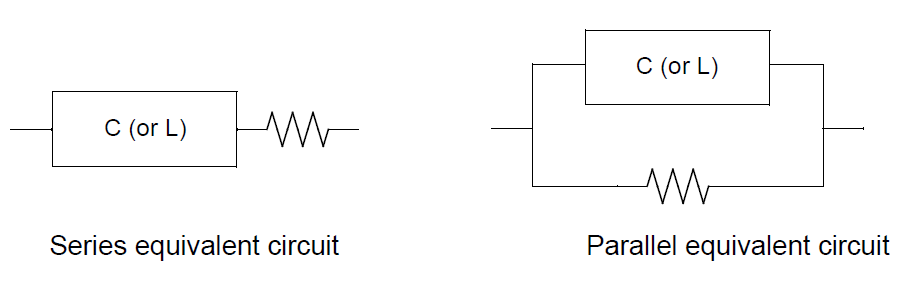# Selecting the equivalent circuit mode of the LCR meter

## QWhen measuring capacitors and inductance with an LCR meter, which should I choose? - Series equivalent circuit mode or Parallel equivalent circuit mode?

A

Generally, the series equivalent circuit mode is used when measuring low impedance components (approx. 100 Ω or less) such as large capacitors and low inductance.
The parallel equivalent circuit mode is used when measuring high impedance components (approx. 10 kΩ or more) such as low capacitors or high inductance.
If the equivalent circuit mode is unknown, such as an impedance of approx. 100 Ω to 10 kΩ, please check with the component manufacturer.

Hioki's LCR Meter determines parameters Z and θ by measuring the current flowing to the test sample and the voltage at both ends of the test sample. Other measurement parameters such as L, C, and R are calculated from Z and θ.
In this case, the mode of calculation assuming that the resistive component exists in series with respect to C (or L) is the series equivalent circuit mode.
The parallel equivalent circuit mode is the mode in which calculations are made assuming that the resistive components exist in parallel with respect to C (or L).
Therefore, since the calculation formulas are different in series equivalent circuit mode and parallel equivalent circuit mode, it is necessary to select the correct equivalent circuit mode to reduce errors.

•